# Dices throws

What is the probability that the two throws of the dice:

a) Six falls even once
b) Six will fall at least once

p1 =  0.6944
p2 =  0.3056

### Step-by-step explanation: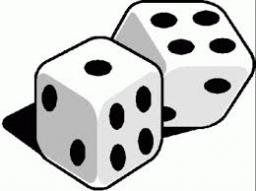Did you find an error or inaccuracy? Feel free to write us. Thank you!Tips to related online calculators
Would you like to compute count of combinations?

## Related math problems and questions:

• Six on diceWhat is the probability that when throwing two dice will fall at least one six?
• Fall sum or sameFind the probability that if you roll two dice, it will fall the sum of 10, or the same number will fall on both dice.
• Sum or productWhat is the probability that two dice fall will have the sum 7 or product 12?
• One threeWe throw two dice. What is the probability that max one three falls?
• Double probabilityThe probability of success of the planned action is 60%. What is the probability that we will achieve success at least once if this action is repeated twice?
• ThrowWe throw 2 times with 2 dices. What is the probability that the first roll will fall more than sum of 9 and the second throw have sum 3 or does not have the sum 4?
• DicesWe will throw two dice. What is the probability that the ratio between numbers on first and second dice will be 1:2?
• Probability of intersectionThree students have a probability of 0.7,0.5 and 0.4 to graduated from university respectively. What is the probability that at least one of them will be graduated?
• DiceWe throw 10 times a playing dice. What is the probability that the six will fall exactly 4 times?
• We rollWe roll two dice A. - what is the probability that the sum of the falling numbers is at most 4 B. - is at least 10 C. - is divisible by 5?
• DiceWe throw five times the dice. What is the probability that six fits exactly twice?
• Hockey players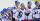After we cycle, five hockey players sit down. What is the probability that the two best scorers of this crew will sit next to each other?
• One dice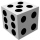Calculate the probability of one dice roll with the numbers 1, 2, 3, 4, 5, 6 on the walls. Write the results in a notebook in the shape of a fraction in the basic form: 2/3. a, The number 1 falls on the cube. b, The number 5 falls on the cube. c, An even
• Dice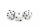How many times must throw the dice, the probability of throwing at least one six was greater than 90%?
• The diceWhat is the probability of events that if we throw a dice is rolled less than 6?
• Toys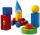3 children pulled 12 different toys from a box. Many ways can be divided toys so that each child had at least one toy?
• Round destiny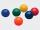There are 5 white and 10 red balls in the destiny. 4 balls will be drawn at random. What is the probability of the event "at least 2 spheres are white"?